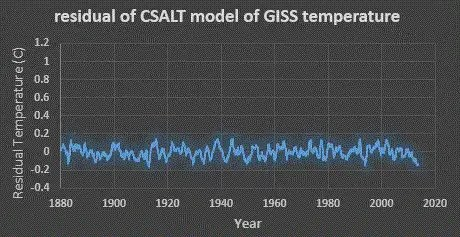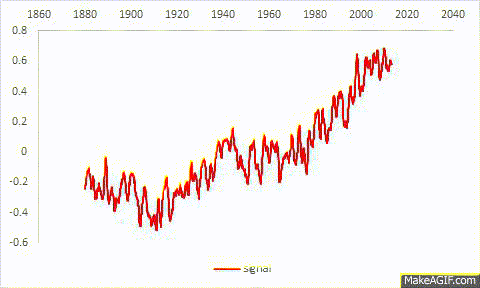# Variational Principles in Thermodynamics

James Annan: “There’s so little interesting stuff going on in climate science these days.”

… well of course, if we don’t have anything worthwhile to say.   But then we also find this:

Peter Ván: “The basic mystery in thermodynamics is the universality. The validity of thermodynamic equations and theories regularly exceed the expectations.” 

The CSALT model of the global temperature anomaly has no right to work as well as it does. After all, it solves no dynamical behavior and requires little information with regards to the complexity of the earth’s surface. Yet, it still captures all the useful detail in the historical temperature record, leaving behind a residual close to being in the white noise regime.Fig. 1 : Residual noise of the CSALT model is flat and close to white noise

The original post on the CSALT model gave a flavor of the variational approach that I used — essentially solving for a thermostatic free energy minimum with respect to the constitutive parameters available. But since the approach has likely been applied before, I deemed it worthwhile to dig for a good fundamental paper from the literature which explains the model in terms of more formal physics.

“Variational Principles in Thermodynamics” by W. Muschik, P. Ván, and C. Papenfuss  (hereafter referred to as MVP) covers the ground in a way that fits the problem space to a tee.   The premise of the MVP paper is that the variational approaches used in ordinary structural mechanics, which typically use an energy Hamiltonian to solve problems (such as the arc of a suspended catenary cable),  could also be applied to solve thermodynamic problems. The caveat being that certain preconditions must be met for it to be cast as an extremal problem:

Abstract: “Dynamic behavior in physics is mostly described by equations of motion which form a system of ordinary or partial differential equations describing the evolution of the physical system in consideration in space and time.

By presupposing sufficient conditions an evolution criterion can be derived from the Second Law which results in a Hamiltonian variational principle, also in thermodynamics.”

If the sufficient conditions are met, the advantage of the variational approach is that differential equations describing the equivalent Hamiltonian equations do not have to be solved. Instead, a more elegant solution drops out of the constraints via the calculus of variations.

“In general the governing equations of non-equilibrium thermodynamics, that are the balance equations of mass, momentum, angular momentum, energy, entropy, and others for additional fields describing the system in consideration, are not variational self-adjoint. Consequently a Hamiltonian variational problem for non-equilibrium thermodynamics is not available. That is just the reason why people use procedures described in section 3. All these procedures are mathematically induced: One wants to formulate a non-Hamiltonian variational problem, whatever the reasons for that may be. Here now we want to treat the more physically induced problem: Starting out with the Second Law, what are the physical presuppositions we have to make, so that we can formulate a variational problem?

We will see that this aim can be reached in two different steps. First of all we derive from the Second Law in form of the global balance for the entropy.”

MVP’s derivation starts with the following global entropy balance equation capturing the flow of entropy produced (1) internally, (2) from an entropy field(s), and (3) from an entropy supply.

$$frac{d}{dt}int_{G(t)}rho S dV + oint_{partial G(t)} Phi cdot dF – int_{G(t)}rho phi dV ge 0$$

They then suggest that a valid evolution criteria for G(t) (as the body out of equilibrium) has to take a form similar to the first term above (we will see this in the next equation).  Then the values for mass density ρ, specific entropy field S, entropy flux density field Φ, and the entropy supply φ, form a variational problem in which the integral is maximal under equilibrium.

After reducing the abstract entropy flux terms to concrete constitutive variables, we get the following relation:

$$frac{d}{dt}int_{G(t)} left[ {rho T S} – {rho epsilon} – {rho gamma} – P – {frac{1}{2} rho v^2} right] dV ge 0$$

This integrand is a special kind of negative free energy modified by the equilibrium pressure (P) and  kinetic energy (~ v^2) of the environment.   Since this does not need the hypothesis of local equilibrium (as the Gibbs fundamental equation was not used) it remains valid during the entire non-equilibrium process.

The sufficient conditions that MVP assert as evolution criteria include:

• There is no entropy- and energy-supply (i.e. φ is zero)
• The entropy flux density through the contour integral is purely thermal
• The force density is conservative
• The environment of the system has reservoir properties, that means it is always in equilibrium and its state is time independent (T and P are constant)
• The vicinity consists of a perfect fluid.

The final equation represents a modified negative free energy of the system G(t).   MVP state that “The integral in the evolution criteria is maximal in equilibrium and consequently constitutes a variational principle.”

$$delta int_{G(t)} {mathcal F}(x, t rightarrow infty) dV = 0$$

where

$${mathcal F} equiv {rho T S}-{rho epsilon}-{rho gamma}-P-{frac{1}{2} rho v^2}$$

MVP make an essential point that the evolution criteria can be derived from the Second Law by sufficient assumptions without any additional constraints.

In practical terms, we make the association between the variational terms and observational measurements of the earth’s troposphere.

$${rho T S} rightarrow$$  Temperature and Heat Capacity

$${rho epsilon}rightarrow$$  LOD as internal energy

$${rho gamma}rightarrow$$  CO2, Aerosols, TSI as external energy

$${P}rightarrow$$  SOI as Pressure

$$frac{1}{2}rho v^2 rightarrow$$  Atmospheric Angular Momentum (AAM) as kinetic energy

The important point about this free energy formulation is that these factors must exist in the atmosphere as they describe obvious observable and measurable characteristics.  The only question is the magnitude of the effects given the  natural variation that exists as fluctuation phenomenon.Fig 2: The CSALT model as a free energy variational approach isolates the “nuisance” constitutive factors leaving behind the residual noise and the factor of interest, in this case the ln(CO2) forcing.

In a thread discussing the Cotwan and Way paper :

Nick Stokes: GCM’s solve the Navier-Stokes equations, with transport of materials and energy, and of course radiation calculations. A GCM requires as input a set of forcings, which depend on scenario. GISS forcings are often cited. But a model does not use as input any temperature record.

This is a valuable point.  The CSALT model when describing the temperature record does not actually use temperature as input other than as a variable in the free energy formulation as described above.  The model performs as a variational short-cut to the more complex General Circulation Models (GCM) used to do the detailed simulations. And as a way to reveal the important factors, such as determining the CO2 contribution to global warming, it is an extremely valuable tool.

## References

 P. Ván, “Thermodynamics of continua: the challenge of universality,” arXiv preprint arXiv:1305.3582, 2013.
 W. Muschik, P. Ván, and C. Papenfuss, “Variational principles in thermodynamics,” Technische Mechanik, vol. 20, no. 2, pp. 105–112, 2000. http://www.ovgu.de/ifme/zeitschrift_tm/2000_Heft2/Muschik.pdf
 K. Cowtan and R. G. Way, “Coverage bias in the HadCRUT4 temperature series and its impact on recent temperature trends,” Q.J.R. Meteorol. Soc., p. n/a–n/a, Nov. 2013.

## 2 thoughts on “Variational Principles in Thermodynamics”

1.admin

This is a fun criticism I found of the CSALT technique and my response

NW | November 21, 2013 at 2:28 am |

You can’t be bothered to look into simultaneous equations bias, or the meaning of the regression assumption E(e|X) = 0, or the spurious regression phenomenon that crops up when using time series data. None of this is likely to undermine your model completely, but learning about it would help you refine your approach to the naturally occurring data (which you don’t encounter in your lab, where you have all kinds of control), get more defensible estimates, plausibly interpret them in a causal way and so forth. I am certain that, at one time, you were educable, but many people lose that somewhere along their way.”

This is the analogy. I am looking at the response function of an electrical circuit that consists of various components. These are all arranged in some configuration and obey the physics of current flow, such as Ohm’s Law. By doing a regression of the measured output current against time, I can easily come up with estimates of the unknown parameters. That is all I am doing for now.

I am not looking into “simultaneous equations bias” or “spurious regression” because what I am doing is trying to provide some insight as to what is happening.

If you want to be of some help, why don’t you find something wrong with the modified negative free energy formulation that W. Muschik, P. Ván, and C. Papenfuss define as the thermodynamics of the system.

Be specific and we can make it better. Just don’t take some thinly veiled potshots that only serve to create FUD.

Like